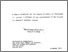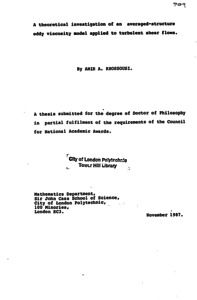# A theoretical investigation of an averaged-structure eddy viscosity model applied to turbulent shear flows

Khossousi, Amir A. (1987) A theoretical investigation of an averaged-structure eddy viscosity model applied to turbulent shear flows. Doctoral thesis, City of London Polytechnic.Preview
Text
378944.pdf - Published Version

## Abstract / Description

The project is concerned with the study of a new mathematical model in which the structure of turbulence is described by averaging the effects of the eddies of various scales over the whole volume of flow. The model assumes that the turbulent stresses can be presented in a way analogous to the laminar stresses and the correlations between the point in question with those at other locations in the flow follow a distribution which may be assumed Gaussian.

The model is initially applied to the steady incompressible turbulent Poiseuille flows between parallel flat plates and through circular pipes. The Navier-Stokes equations are simplified and solved analytically, with the aid of Integral Transform methods and asymptotic expansions. With the appropriate numerical values for the model constants, the approximate asymptotic solutions for the mean velocities are found in good agreement with the universal laws and with the experimental data.

To test the model further, the steady incompressible turbulent boundary layer flow along the surface of a solid body is studied. It is shown that under the similarity transformations the boundary-layer equations may be simplified to give, to the first approximation, ordinary differential equations of order three. These equations are similar to the Blasius and the Falkner-Skan equations except for the presence of the additional terms representing' the turbulent effects.

With the usual no-slip conditions at the wall, numerical solutions to these non-linear equations are obtained for various values of the flow parameters. For the special case when the pressure gradient related parameter n=-1, it is described how an approximate analytic solution can be obtained, by extending the existing solutions of the Falkner-Skan equation.

Item Type: Thesis (Doctoral) uk.bl.ethos.378944 turbulent flow equations; mathematical models; Falkner-Skan equations 500 Natural Sciences and Mathematics > 510 Mathematics Library Services and Special Collections Mary Burslem 13 May 2022 15:14 13 May 2022 15:14 https://repository.londonmet.ac.uk/id/eprint/7628View Item